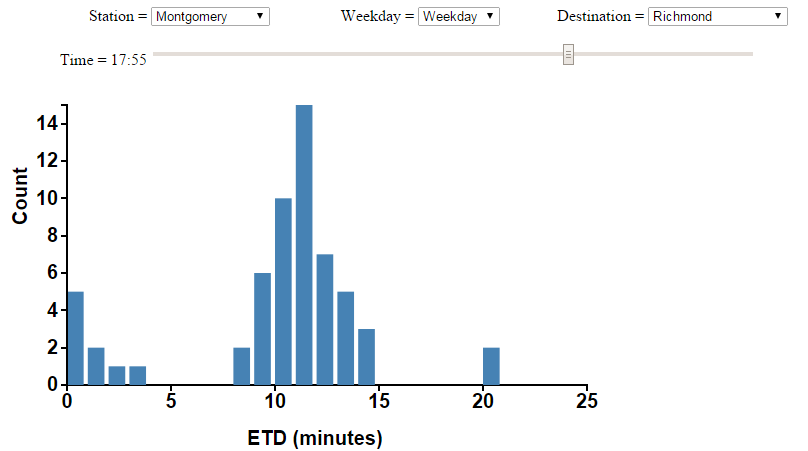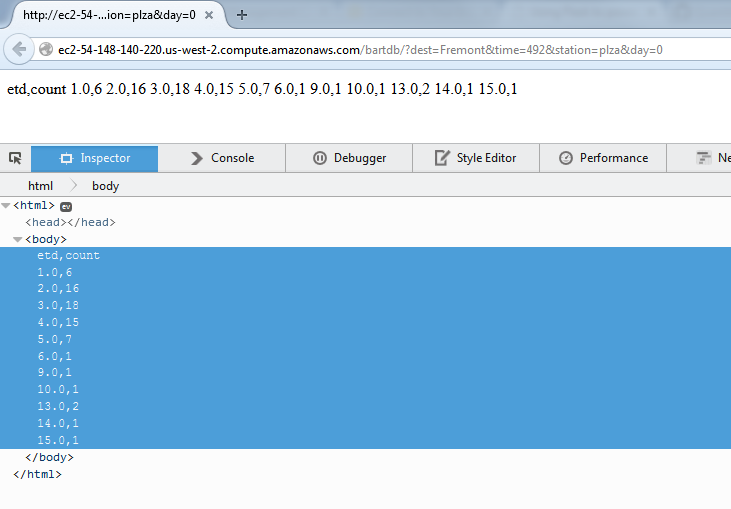# Getting csv data from requests to a SQL backed Flask app

1. Running a Flask app on AWS EC2
3. Getting csv data from requests to a SQL backed Flask app

In this post I'll describe a Flask app that receives requests from a D3.js plotting script and returns csv format data from a SQL database. See part 1 and part 2 for background information.

Source code for database creation, Flask app

# The dataAbove is what our end result looks like when plotted. Any selection for what data to graph gets sent to the Flask server and the selected data is returned from the SQL database.

The data is acquired from the BART API, after a bit of xml parsing I create csv files with data about the each train's ETD, destination, etc (see `plza.csv`, related post).

```\$ head -n5 plza.csv
time,dest,dir,len,etd
1417108837.85,Fremont,South,6,3
1417108837.85,Richmond,North,6,10
1417108921.12,Fremont,South,6,2
1417108921.12,Richmond,North,6,9
```

# Creating the SQL database

The script below reads in the data from the csv files and ouputs it into a SQL database, using pandas to do a lot of the work.

```"""
Read in BART ETD data from files and write to SQL.
"""
import sqlite3
import pandas as pd
import numpy as np

DATABASE = 'bart.db'
FILES = ['plza.csv', 'mont.csv']

def parse_time(timestamp):
"""Attempt to parse a timestamp (in seconds) into a pandas datetime in
Pacific time, return the timestamp is parsing is successful, NaT (not a
time) otherwise

:return: Pandas timestamp in Pacific time, or NaT
"""
try:
dt = pd.to_datetime(float(timestamp), unit='s')
return dt.tz_localize('UTC').tz_convert('US/Pacific')
except (AttributeError, ValueError):
return pd.NaT

def define_weekday(obs_time):
"""Return 0 if obs_time occurred on a weekday, 1 if a Saturday, 2 if a
Sunday.

:param obs_time: pandas timestamp
"""
if obs_time.weekday() < 5:
return 0
elif obs_time.weekday() == 5:
return 1
elif obs_time.weekday() == 6:
return 2

def parse_data(file_name, date_parser=parse_time, time_col=['time']):
"""Return a dataframe from csv file, with times parsed.

:param file_name: csv file
:param date_parser: function to convert time_col to datetime (default:
parse time)
:param time_col: the time of the column to parse as times
:return: DataFrame from csv file
"""

def time2minute_of_day(obs_time):
"""Return the minute of day (12:00 midnight = 0) of observation time

:param obs_time: pandas datetime object
"""
return obs_time.time().hour * 60 + obs_time.time().minute

def csv2sql(conn, files):
"""Read in BART ETD data from files and write that data to the SQL database
accessed by conn.

:param conn: SQL database connection
:param files: the files to read data from
"""
output_cols = ['dest', 'dir', 'etd', 'station', 'minute_of_day',
'day_of_week']
conn.execute("DROP TABLE IF EXISTS etd")
for sta_file in files:
df = parse_data(sta_file)
df['station'] = sta_file.split('.')
df['day_of_week'] = df['time'].apply(lambda x: define_weekday(x))
df['etd'] = df['etd'].replace('Leaving', 0).dropna().astype(np.int)
df['minute_of_day'] = df['time'].apply(time2minute_of_day)
df[output_cols].to_sql('etd', conn, index=False, if_exists='append')

conn.cursor().execute(
"""CREATE INDEX idx1
ON etd(station, dest, minute_of_day, day_of_week)
"""
)
conn.commit()
conn.close()

if __name__ == '__main__':
conn = sqlite3.connect(DATABASE)
csv2sql(conn, FILES)
```

Creating an index on the variables we will be querying against is key for the performance of our app. The simple `CREATE INDEX idx1 ON etd(station, dest, minute_of_day, day_of_week)` improves the speed of the query below by two orders of magnitude.

To return data for the graph, we need to select data points from trains arriving at a given station in a given direction on a given day of the week at a given time, and make a histogram of the ETD values for those data points. Add the following function to Flask app from part 2 to return this data from our SQL database. You can point your browser at `(my public DNS)/?dest=Fremont&time=12:17&station=plza&day=0` to get csv formatted data ready to pass into D3.js for graphing.

```@app.route("/")
def print_data():
"""Respond to a query of the format:
myapp/?dest=Fremont&time=600&station=plza&day=0
with ETD data for the time and location specified in the query"""
start_time = time.time()
cur = get_db().cursor()
try:
minute_of_day = int(request.args.get('time'))
except ValueError:
return "Time must be an integer"
station = request.args.get('station')
print minute_of_day
day = request.args.get('day')
dest = request.args.get('dest')
result = execute_query(
"""SELECT etd, count(*)
FROM etd
WHERE dest = ? AND minute_of_day = ?
AND station = ? AND day_of_week = ?
GROUP BY etd""",
(dest, minute_of_day, station, day)
)
str_rows = [','.join(map(str, row)) for row in result]
query_time = time.time() - start_time
logging.info("executed query in %s" % query_time)
cur.close()
```

Here is an example query and result, along with Firefox inspector output showing the csv format more clearly:This is the data our D3.js visualization will act on in Part 4.

1. Running a Flask app on AWS EC2